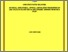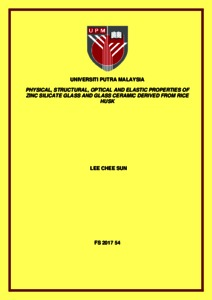# Physical, structural, optical and elastic properties of zinc silicate glass and glass ceramic derived from rice husk

## Citation

Lee, Chee Sun (2017) Physical, structural, optical and elastic properties of zinc silicate glass and glass ceramic derived from rice husk. Masters thesis, Universiti Putra Malaysia.

## Abstract

In this study, fabrications zinc silicate (ZnOSiO₂) system were fabricated using zinc oxide (ZnO) and white rice husk ash (WRHA). Rice husk was burned to form WRHA and was used as a substitution for silica. Compositions of (ZnO)x(WRHA)1-x(x = 0.55, 0.60, 0.65 and 0.70 wt.%) was labeled S1, S2, S3 and S4 using the melt-quench method. First of all, the chemical composition of the samples fabricated has been analyzed using Energy Dispersive X-ray Fluorescence (EDXRF) technique. Its physical properties have been measured with density through Archimedes principles and molar volume. Density of the samples increases from 2.94 to 3.66 g cm-3 while the molar volume decreases from 23.97 to 20.18 cm3 mol-1 when the amount of ZnO in the samples increases. Next, structural properties of the samples have been analyzed using X-Ray Diffraction (XRD) and Fourier Transform Infrared (FTIR) Spectroscopy. XRD shows that not all samples produced are in amorphous state and only sample S1 is in amorphous while samples S2, S3 and S4 is in crystalline as the amount of ZnO increases. FTIR results shows that non-bridging oxygens (NBOs) are formed as ZnO increase in the samples. Optical properties of the samples have been analyzed using UV-Visible Spectroscopy (UV-Vis). As the ZnO in the 2 samples increase, the absorption increases with the increase of crystallization and shifts to higher wavelength. Optical band gap of the samples decreases with the increase in ZnO as NBOs increases. Optical band gap of the samples arises with Eopt values for n = 3/2 after comparing the value from the exitation coefficient and differential curve with various number of n. The optical band gap Eopt values for n = 3/2 decreases from 4.50 to 4.41 eV as ZnO increases. Ultrasonic velocity of the samples was used to calculate the elastic properties of the samples. Decrease of longitudinal and shear velocity was 3857.03 to 2482.31 ms-1 and 1951.95 to 966.27 ms-1 respectively. Elastic moduli such as longitudinal modulus, shear modulus, Young modulus, bulk modulus, Poisson’s ratio, fractal bond connectivity and Micro-hardness of the samples have been calculated. All the elastic moduli decrease except for Poisson’s ratio. Elastic moduli are an important tool to measures sample’s resistance to be deformed elastically or permanently. Elastic moduli such as longitudinal modulus, shear modulus, Young modulus, bulk modulus, Poisson’s ratio was compared with the theoretical elastic moduli from Rocherulle’s model. Comparison of both of these elastic moduli shows a similar trend in longitudinal modulus, shear modulus, Young modulus and bulk modulus except for the Poisson’s ratio.Preview
Text
FS 2017 54 IR.pdfView Item